# 2 Motivating Examples

Latex is best known for typesetting math equations. However, many packages have been developed to create other difficult to typeset elements including plots, chemical equations, multi-lingual documents, and music. Below are examples of some of the applications of Latex.

# Mathematics

Suppose we want to solve the general quadratic equation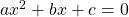. If you isolate for x, you derive the well-known quadratic formula: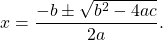The default distribution of Latex offers over 10,000 symbols. So, you can easily typeset most equations: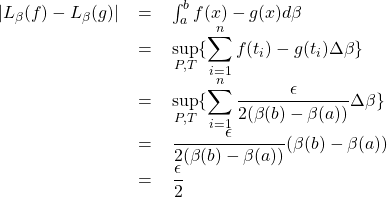# Chemical Equations: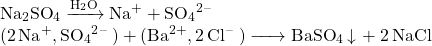Credit: Stack Exchange

# Phonetic Transcription: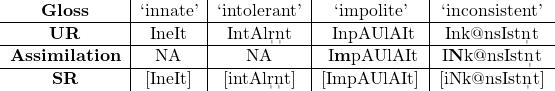# Music: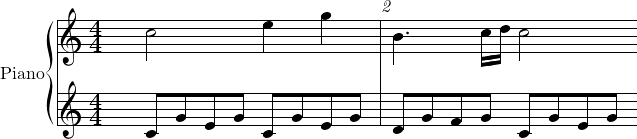Credit: Musixtex Documentation

# Plots: